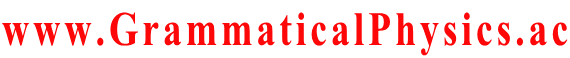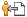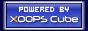Welcome SourceCodeOf_HumanGenome
www.GrammaticalPhysics.ac
Account
Access Detector
1 user(s) are online (1 user(s) are browsing Forum)

Members: 1
Guests: 0

SourceCodeOf_HumanGenome more...

# Another Type of an Entangled Solution

SourceCodeOf_HumanGenome > Another Type of an Entangled Solution @ 2013/4/29 15:15
Φ[χ] ＝ Σp,q Πj ∫dxj ∫dtj G(χ(pj), pj; xj, tj)F(xj, tj)G(χ(qj), qj; xj, tj)
where I divided the set {1/α, 2/α, 3/α, ・・・} into pairs {(p1, q1), (p2, q2), (p3, q3), ・・・}.
Σp,q means the sum over all patterns of division.

I expect that the path integral:
j≠j0 and j≠j1 ∫dχ(j/α)]Φ[χ]*Φ[χ]
yields some function resembling G(χ(j0/α), j0/α; χ(j1/α), j1/α) when F(x, t) = 1.

∫dx G(x1, t1; x, 0)G(x2, t2; x, 0) resembles G(x1, t1; x2, t2) because G(x2, t2; x, 0) represents the wavefunction Ψ(x, 0) such that Ψ(x, t2) = δ(x - x2).

G(x2, t2; x, 0) = ＜x2|U(t2, 0)|x＞
= ＜x|U(t2, 0)|x2*
= ＜x|U(t2, 0)-1|x2*
= ＜x|U(0, t2)|x2*
= G(x, 0; x2, t2)*
This is the Green's function of Ψ*.

Ψ* obeys the time reversed Schrodinger equation, but it is essentialy the same as Ψ when Ψ(x, t2) = δ(x - x2).
So,
∫dx G(x1, t1; x, 0)G(x2, t2; x, 0)
= ∫dx'∫dx G(x1, t1; x, 0)G(x', t2; x, 0)Ψ*(x', t2)
is essentially the same as
∫dx'∫dx G(x1, t1; x, 0)G(x, 0; x', t2)Ψ(x', t2)
= ∫dx' G(x1, t1; x', t2)Ψ(x', t2)
= G(x1, t1; x2, t2)
where Ψ(x', t2) = δ(x' - x2).

---
Last edited at 2013/05/01/15:29JST

Category
Forum
Global

Notification method is private message [Change]

Site Search
Site Info
WebmasterswooderRecommend Us